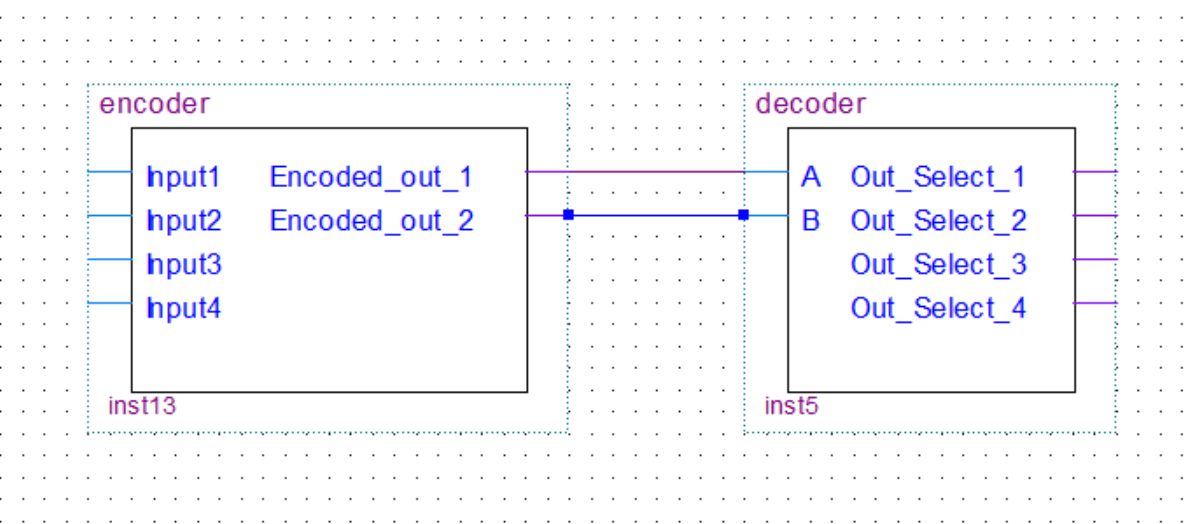# 8-Bit Computer Encoders and DecodersShare:

In my 8-bit computer build, I only used decoders. However, I will still cover encoders in this post for the sake of completeness. You can see the decoders being used in the RAMmultiplexers, and the control unit of the computer.

Decoders decode the binary value on the input to an individual selection on the output. The encoder will of course do the exact opposite.

In this post, we’ll take a look at encoders/decoders with 4 inputs/outputs because it’s easier to understand how they work. The logic is the same for more inputs/outputs, the logic circuit just gets scaled up.

## Decoder

In this example, we have a 2 to 4 decoder(described as n to 2). The n stands for the number of input lines. This decoder will take 2 input lines in and decode(select an output by setting it high) them to 2n or in this case 4 output lines.

We start out with two inputs(A and B). Because we are dealing with digital logic we have binary values. So two values and two inputs give us four different combinations(00, 10, 01, 11). To be able to represent all the states we add a NOT gate to each input. This way we have all the possible combinations. So for example if we want the first output(Out_Select_1) to be 1 the two inputs to the AND gate have to be connected in such a way that they will both be 1 when A and B are both 0.
The same thing is done for the other outputs but for a different combination.

### Truth Table

A B Out_1 Out_2 Out_3 Out_4
0 0 1 0 0 0
1 0 0 1 0 1
0 1 0 0 1 0
1 1 0 0 0 1

out_1 = A'.B'
out_2 = A.B'
out_3 = A'.B
out_4 = A.B

## Encoder

The encoder will do the opposite of the decoder. In this example, we have a 4 to 2 encoder(described as 2n to n ). The n stands for the number of output lines. This encoder will take 4 or 2n input lines in and encode them to n or in this case 2 output lines(select an output by setting it high).

### Truth table

Input4 Input3 Input2 Input1 out_2 out_1
0 0 0 1 0 0
0 0 1 0 0 1
0 1 0 0 1 0
1 0 0 0 1 1

### Boolean Expression

out_1 = Input2 + Input4
out_2 = Input3 + Input4
Share: